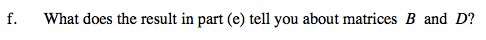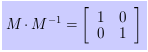### Home > PC > Chapter 12 > Lesson 12.2.1 > Problem12-43

12-43.
1. Given the matrices listed below, complete the operations specified. Homework Help ✎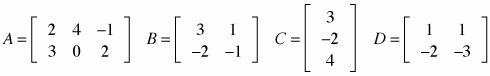1. B + D

2. 2A

3. AC

4. CA

5. BD

6. What does the result in part (e) tell you about matrices B and D?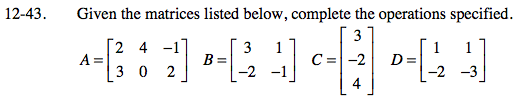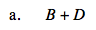$\begin{bmatrix}3+1&1+1\\-2+(-2)&-1+(-3)\end{bmatrix}$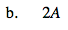Double each entry in matrix A.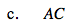Multiply each row in matrix A by the column in matrix C.

$\begin{bmatrix}2(3)+4(-2)+(-1)(4)\\3(3)+0(-2)+2(4)\end{bmatrix}$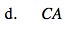Do the rows of matrix C have the same number of entries as the columns of matrix A?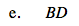Multiply the rows of matrix B by the columns of matrix D.